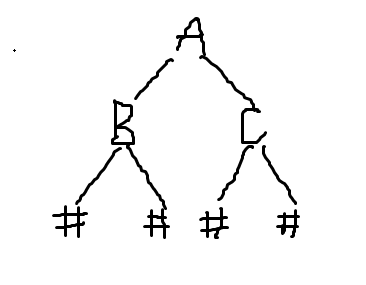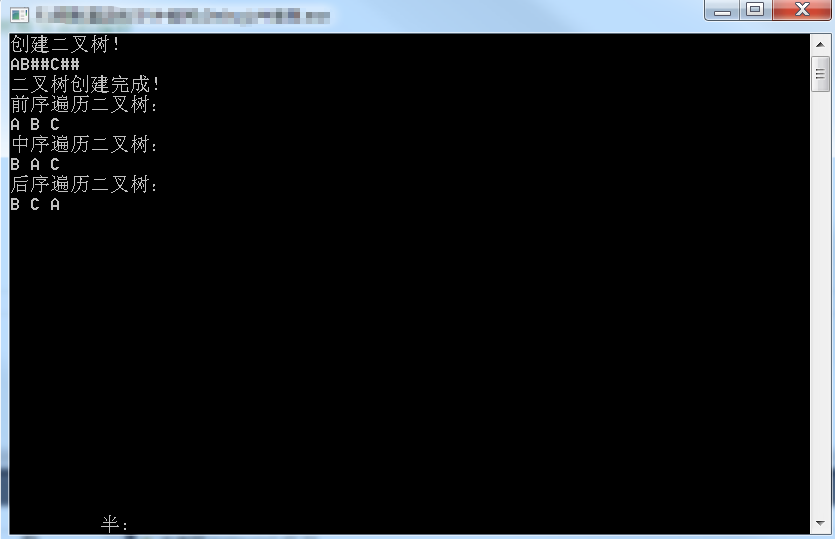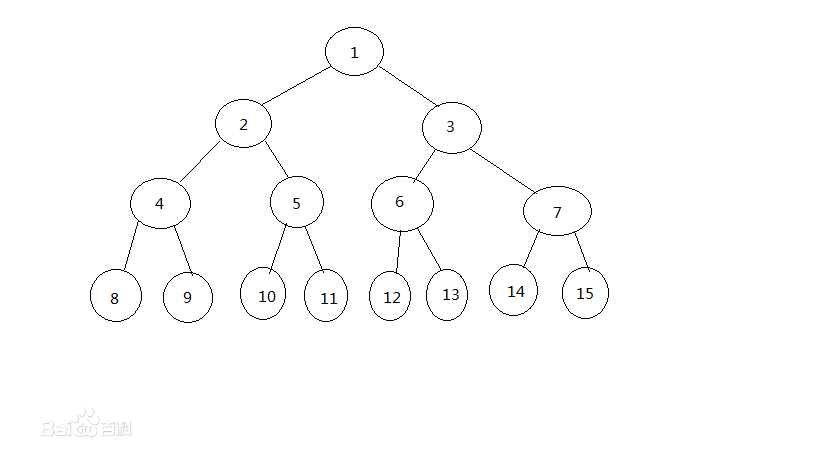• 二叉树建立（C++）
千次阅读 多人点赞
2020-09-08 14:47:14

二叉树的建立、递归遍历、层次遍历

#include<iostream>
#include<queue>
using namespace std;
class tree {
public:
char value;//结点值
tree *liftchild;//左子树指针
tree *rightChild;//右子树指针
};
void creat_tree(tree * &T)//创建二叉树
{
char ch;
ch = getchar();//从键盘输入一串字母,依次读取,直至换行键
if (ch == '#')
T = NULL;
else {
T = new tree;
T->value = ch;
creat_tree(T->liftchild);
creat_tree(T->rightChild);
}
}
void VLR(tree *T)//先序遍历二叉树
{
if (T == NULL)
return;
else {
cout << T->value;
VLR(T->liftchild);
VLR(T->rightChild);
}
}
void LVR(tree *T)//中序遍历二叉树
{
if (T == NULL)
return;
else {
LVR(T->liftchild);
cout << T->value;
LVR(T->rightChild);
}
}
void LRV(tree *T)
{
if (T == NULL)
return;
else {
LRV(T->liftchild);

LRV(T->rightChild);
cout << T->value;
}

}
int caclnum(tree* T)//计算结点个数
{
if (T == NULL)
return 0;
else
{
return 1 + caclnum(T->liftchild) + caclnum(T->rightChild);//计算二叉树结点个数
}

}
void LevelOrder(tree * T)//层次遍历
{
if (T == NULL)
return;
queue<tree *> deq;//建立一个队列容器

deq.push(T);
while (!deq.empty())
{
tree *tr = deq.front();
cout << tr->value << endl;
deq.pop();
if (tr->liftchild != NULL)
deq.push(tr->liftchild);

if (tr->rightChild!= NULL)
deq.push(tr->rightChild);
}
}
int main() {

tree * T ;
creat_tree(T);
VLR(T);//前序遍历
cout << endl;
LVR(T);//中序遍历
cout << endl;
LRV(T);//后序遍历
cout << caclnum(T);//统计结点个数
LevelOrder(T);//层次遍历
system("pause");

return 0;
}

更多相关内容
• 通过链式存储结构实现二叉树创建，包含如下操作： 1. 创建树； 2. 销毁树； 3. 清空树； 4. 插入结点； 5. 删除结点； 6. 获取结点； 7. 获取根结点； 8. 获取树的结点数； 9. 获取树的高度； 10. 获取树的度； 11...
• 建立二叉树，实现二叉树的先序、中序、后序的递归遍历算法，输出遍历结果。 实现二叉树的先序、中序、后序和层次遍历的非递归算法，输出遍历结果。
• ## 二叉树建立

万次阅读 多人点赞 2019-03-20 20:57:36
结束二叉树输入：如何结束创建二叉树的输入那；把二叉树补全 前序（输入）：AB##C## 中序：#B#A#C# 后序：##B##CA 输出结果如下： 代码如下 #include<iostream> using namespace std; //定义节点 ...

结束二叉树输入：如何结束创建二叉树的输入那；把二叉树补全前序（输入）：AB##C##

中序：#B#A#C#

后序：##B##CA

输出结果如下：代码如下

#include<iostream>
using namespace std;

//定义节点
typedef struct node
{
struct node *left;
struct node *right;
char data;
}BiTreeNode, *BiTree;

//按照前序顺序建立二叉树
void createBiTree(BiTree &T)
{//输入需要将二叉树补成满二叉树  ABD##E##CH###  或者 AB##C##
char c;
cin >> c;
if ('#' == c)             //当遇到#时，令树的根节点为NULL，从而结束该分支的递归
T = NULL;
else
{
T = new BiTreeNode;
T->data = c;
createBiTree(T->left);
createBiTree(T->right);
}
}

//前序遍历输出
void preTraverse(BiTree T)
{
if (T)
{
cout << T->data << " ";
preTraverse(T->left);
preTraverse(T->right);
}
}
//中序遍历输出
void midTraverse(BiTree T)
{
if (T)
{
midTraverse(T->left);
cout << T->data << " ";
midTraverse(T->right);
}
}
//后续遍历输出
void postTraverse(BiTree T)
{
if (T)
{
postTraverse(T->left);
postTraverse(T->right);
cout << T->data << " ";
}
}
int main()
{
BiTree T;               //声明一个指向二叉树根节点的指针
createBiTree(T);
cout << "二叉树创建完成！" << endl;
cout << "前序遍历：" << endl;
preTraverse(T);
cout << endl;
cout << "中序遍历：" << endl;
midTraverse(T);
cout << endl;
cout << "后序遍历：" << endl;
postTraverse(T);
return 0;
}

展开全文• 采用二叉链表存储，实现二叉树创建、遍历（递归）、赫夫曼编码和译码等典型操作。 1. 编程实现如下功能： （1）假设二叉树的结点值是字符型，根据输入的一棵二叉树的完整先序遍历序列（子树空用’#’表示），建立...
• 关于二叉树的一些操作，内容简单易懂，关于二叉树创建遍历等等问题，适合新手
• 1.采用二叉链表作为存储结构，创建一棵二叉树； 2.用递归及非递归算法对二叉树实现先序遍历； 3.用递归及非递归算法对二叉树实现中序遍历； 4.用递归及非递归算法对二叉树实现后序遍历。 5.用递归遍历算法中的访问...
• Java实现二叉树的3种方式，顺序二叉树的实现，二叉树的三叉链表存储，二叉树的二叉链表实现
• ## C语言二叉树创建（一定看的懂）

万次阅读 多人点赞 2018-06-02 14:01:36
先贴一个百度出来的二叉树的图 二叉树 就是首先得有一个根节点.这个节点的入度为0也就是它只有子节点没有父节点 如1号节点     每个节点又有一个左儿子和一个右儿子 当然也可以没有 接下来就是创建.创建一...

先贴一个百度出来的二叉树的图二叉树 就是首先得有一个根节点.这个节点的入度为0也就是它只有子节点没有父节点 如1号节点

每个节点又有一个左儿子和一个右儿子 当然也可以没有

接下来就是创建.创建一棵树得现有这棵树的结点和树根

首先来声明一个树的结点

typedef struct node{ //树的结点
int data;
struct node* left;
struct node* right;
} Node;

当然 int data;这里叫做数据域 也可以多写一些.

然后就是树根了

typedef struct { //树根
Node* root;
} Tree;

树根创建完了然后就可以写创建树的函数了

void insert(Tree* tree, int value)//创建树
{
Node* node=(Node*)malloc(sizeof(Node));//创建一个节点
node->data = value;
node->left = NULL;
node->right = NULL;
}

第一个参数是这个树的树根。想想我们的链表 如果头插法的话是不是把头传到函数里面就可以了

第二个节点是值 也就是 我们之前节点的data

第一行动态申请一个空间因为是malloc 出来的所以在堆内存中是有实例的

不会随着函数结束而释放

创建出来以后让左儿子和右儿子为null 让指针初始化的时候指向NULL是一个很好的习惯

然后结点创建出来就要把它连到树里面了。

如果这个树是空树我是不是可以让树根存下这个节点呢？

if (tree->root == NULL)//判断树是不是空树
{
tree->root = node;
}

如果不是空树的话我们可以给一个约束条件

我们规定这个树的父节点小于右儿子又大于左儿子 也就是

左儿子 < 父节点 < 右儿子。

我们先按照这样去约束

所以再树已经不是空树的时候我们就可以从头开始判断

else {//不是空树
Node* temp = tree->root;//从树根开始
while (temp != NULL)
{
}

小于进左儿子 大于进右儿子

不管进了哪一边我们无非就两种情况有节点或者是NULL

是NULL我们直接让新建的节点就放在这就可以了 然后返回对吗

如果有节点呢？

那我们应该再判断一遍，还是和之前一样。

只要一和前面有重复的操作那我们架个循环跑就好了 对不对

void insert(Tree* tree, int value)//创建树
{
Node* node=(Node*)malloc(sizeof(Node));//创建一个节点
node->data = value;
node->left = NULL;
node->right = NULL;
if (tree->root == NULL)//判断树是不是空树
{
tree->root = node;
}
else {//不是空树
Node* temp = tree->root;//从树根开始
while (temp != NULL)
{

if (value < temp->data)//小于就进左儿子
{
if (temp->left == NULL)
{
temp->left = node;
return;
}
else {//继续判断
temp = temp->left;
}
}
else {//否则进右儿子

if (temp->right == NULL)
{
temp->right = node;
return;
}
else {//继续判断
temp = temp->right;
}
}
}
}
return;
}

好了 创建的函数结束了

然后遍历二叉树 一般用中序遍历

因为用中序遍历可以从小到大去遍历如果不懂的可以去查一下

好了来一发完整代码

#include <cstdlib>
#include <stdio.h>

typedef struct node{ //树的结点
int data;
struct node* left;
struct node* right;
} Node;

typedef struct { //树根
Node* root;
} Tree;

void insert(Tree* tree, int value)//创建树
{
Node* node=(Node*)malloc(sizeof(Node));//创建一个节点
node->data = value;
node->left = NULL;
node->right = NULL;
if (tree->root == NULL)//判断树是不是空树
{
tree->root = node;
}
else {//不是空树
Node* temp = tree->root;//从树根开始
while (temp != NULL)
{

if (value < temp->data)//小于就进左儿子
{
if (temp->left == NULL)
{
temp->left = node;
return;
}
else {//继续判断
temp = temp->left;
}
}
else {//否则进右儿子

if (temp->right == NULL)
{
temp->right = node;
return;
}
else {//继续判断
temp = temp->right;
}
}
}
}
return;
}

void inorder(Node* node)//树的中序遍历
{
if (node != NULL)
{
inorder(node->left);
printf("%d ",node->data);
inorder(node->right);
}
}

int main()
{
Tree tree;
tree.root = NULL;//创建一个空树
int n;
scanf("%d",&n);
for (int i = 0; i < n; i++)//输入n个数并创建这个树
{
int temp;
scanf("%d",&temp);
insert(&tree, temp);
}
inorder(tree.root);//中序遍历
getchar(); getchar();
return 0;
}

未经允许，不得转载

展开全文C语言 二叉树 C语言二叉树
• 二叉树建立遍历，输入后可以输出二叉树的中序和后序遍历。
• 主要介绍了python使用递归的方式建立二叉树，文中通过示例代码介绍的非常详细，对大家的学习或者工作具有一定的参考学习价值，需要的朋友们下面随着小编来一起学习学习吧
• ## c++二叉树创建

万次阅读 多人点赞 2019-03-03 21:17:48
先贴一个百度出来的二叉树的图 二叉树 就是首先得有一个根节点.这个节点的入度为0也就是它只有子节点没有父节点 如1号节点 每个节点又有一个左儿子和一个右儿子 当然也可以没有 接下来就是创建.创建一棵树...

先贴一个百度出来的二叉树的图二叉树 就是首先得有一个根节点.这个节点的入度为0也就是它只有子节点没有父节点 如1号节点

每个节点又有一个左儿子和一个右儿子 当然也可以没有

接下来就是创建.创建一棵树得现有这棵树的结点和树根

首先来声明一个树的结点

typedef struct node{ //树的结点
int data;
struct node* left;
struct node* right;
} Node;


当然 int data;这里叫做数据域 也可以多写一些.

然后就是树根了

typedef struct { //树根
Node* root;
} Tree;

树根创建完了然后就可以写创建树的函数了

void insert(Tree* tree, int value)//创建树
{
Node* node=(Node*)malloc(sizeof(Node));//创建一个节点
node->data = value;
node->left = NULL;
node->right = NULL;
}

第一个参数是这个树的树根。想想我们的链表 如果头插法的话是不是把头传到函数里面就可以了

第二个节点是值 也就是 我们之前节点的data

第一行动态申请一个空间因为是malloc 出来的所以在堆内存中是有实例的

不会随着函数结束而释放

创建出来以后让左儿子和右儿子为null 让指针初始化的时候指向NULL是一个很好的习惯

然后结点创建出来就要把它连到树里面了。

如果这个树是空树我是不是可以让树根存下这个节点呢？

if (tree->root == NULL)//判断树是不是空树
{
tree->root = node;
}

如果不是空树的话我们可以给一个约束条件

我们规定这个树的父节点小于右儿子又大于左儿子 也就是

左儿子 < 父节点 < 右儿子。

我们先按照这样去约束

所以再树已经不是空树的时候我们就可以从头开始判断

else {//不是空树
Node* temp = tree->root;//从树根开始
while (temp != NULL)
{
}

小于进左儿子 大于进右儿子

不管进了哪一边我们无非就两种情况有节点或者是NULL

是NULL我们直接让新建的节点就放在这就可以了 然后返回对吗

如果有节点呢？

那我们应该再判断一遍，还是和之前一样。

只要一和前面有重复的操作那我们架个循环跑就好了 对不对

void insert(Tree* tree, int value)//创建树
{
Node* node=(Node*)malloc(sizeof(Node));//创建一个节点
node->data = value;
node->left = NULL;
node->right = NULL;
if (tree->root == NULL)//判断树是不是空树
{
tree->root = node;
}
else {//不是空树
Node* temp = tree->root;//从树根开始
while (temp != NULL)
{

if (value < temp->data)//小于就进左儿子
{
if (temp->left == NULL)
{
temp->left = node;
return;
}
else {//继续判断
temp = temp->left;
}
}
else {//否则进右儿子

if (temp->right == NULL)
{
temp->right = node;
return;
}
else {//继续判断
temp = temp->right;
}
}
}
}
return;
}

好了 创建的函数结束了

然后遍历二叉树 一般用中序遍历

因为用中序遍历可以从小到大去遍历如果不懂的可以去查一下

好了来一发完整代码

#include <cstdlib>
#include <stdio.h>

typedef struct node{ //树的结点
int data;
struct node* left;
struct node* right;
} Node;

typedef struct { //树根
Node* root;
} Tree;

void insert(Tree* tree, int value)//创建树
{
Node* node=(Node*)malloc(sizeof(Node));//创建一个节点
node->data = value;
node->left = NULL;
node->right = NULL;
if (tree->root == NULL)//判断树是不是空树
{
tree->root = node;
}
else {//不是空树
Node* temp = tree->root;//从树根开始
while (temp != NULL)
{

if (value < temp->data)//小于就进左儿子
{
if (temp->left == NULL)
{
temp->left = node;
return;
}
else {//继续判断
temp = temp->left;
}
}
else {//否则进右儿子

if (temp->right == NULL)
{
temp->right = node;
return;
}
else {//继续判断
temp = temp->right;
}
}
}
}
return;
}

void inorder(Node* node)//树的中序遍历
{
if (node != NULL)
{
inorder(node->left);
printf("%d ",node->data);
inorder(node->right);
}
}

int main()
{
Tree tree;
tree.root = NULL;//创建一个空树
int n;
scanf("%d",&n);
for (int i = 0; i < n; i++)//输入n个数并创建这个树
{
int temp;
scanf("%d",&temp);
insert(&tree, temp);
}
inorder(tree.root);//中序遍历
getchar(); getchar();
return 0;
}

---------------------
作者：TheSevenSky
来源：CSDN
原文：https://blog.csdn.net/qq_42011541/article/details/80547098

展开全文• C实现二叉树建立及先中后序的遍历，控制台程序，在各版本vs上均可运行
• ## 二叉树建立及遍历

千次阅读 2018-05-25 22:22:16
本来想做纯二叉树的遍历的，但是做的时候感觉加上哈夫曼编码的建立规则吧，于是这个四不像便产生啦。。。不过基本的三种遍历方法还在。求节点数，树的深度，二叉树每层节点数也有的。#include&lt;stdio.h&gt...
• 代码的主要功能包括：二叉树创建、销毁、计算二叉树的深度、递归前序遍历二叉树、非递归前序遍历二叉树、递归中序遍历二叉树、非递归中序遍历二叉树、递归后序遍历二叉树、非递归后序遍历二叉树、递归层次遍历...
• C++创建二叉树及操作包括前序遍历后序遍历层序遍历 深度 叶子节点
• 1.广义表创建二叉树 比如：A(B(,G),C(D(F),E)) 2.二叉树输出广义表
• 10个数据结构课程设计实例二叉树建立遍历冒泡排序快速排序等下载.zip 10个数据结构课程设计实例二叉树建立遍历冒泡排序快速排序等下载.zip 10个数据结构课程设计实例二叉树建立遍历冒泡排序快速排序等下载.zip 10个...
• 编一个程序，读入用户输入的一串先序遍历字符串，根据此字符串建立一个二叉树（以指针方式存储）。 例如如下的先序遍历字符串： ABC##DE#G##F### 其中“#”表示的是空格，空格字符代表空树。建立起此二叉树以后，再...
•c++
• 链表实现二叉树创建和遍历，三种遍历方式实现，二叉树
• 文章目录二叉树的基本概念二叉树的性质(特性)二叉树的节点表示以及二叉树创建1.二叉树的节点表示2.二叉树创建 二叉树的基本概念 二叉树是每个节点最多有两个子树的树结构。通常子树被称作“左子树”（left ...python 数据结构
•数据结构
• ## 二叉树的创建

千次阅读 2021-03-13 11:48:22
二叉树创建代码: node* Tree::creat(node* root) { char ch = _getch();//输入该节点的信息 if (ch == '#')//表示该节点为空 { root = NULL; } else { root = new node; root->data = ch; //生成...
•研究论文
• 主要介绍了C语言排序方法，包含10种排序，数据结构课程设计实例二叉树建立遍历冒泡排序快速排序_二叉排序树_二叉树层次遍历_二叉树非递归遍历_二叉树建立括号匹配直接插入选择代码大学生本科毕业设计期末作业排序...
• 建立二叉树 创建结点 #include<stdio.h> #include<malloc.h> #include<stdlib.h> #include<queue> using namespace std;//1 2 4 -1 -1 -1 3 5 -1 -1 -1 int cout=0; typedef struct......